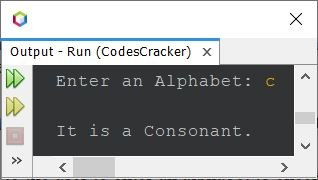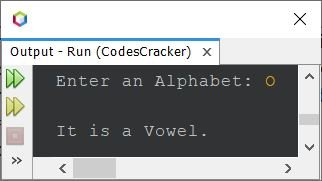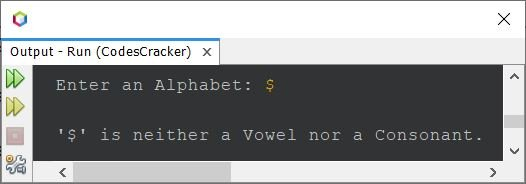# Java Program to Check Vowel or Consonant

This post covers a program in Java that checks whether an alphabet entered by user at run-time of the program, is a vowel or consonant.

Note - a, e, i, o, u, A, E, I, O, U are vowels.

## Check Vowel or Consonant in Java - First Way

The question is, write a Java program to check whether an input character (alphabet) is a vowel or consonant. The program given below is its answer:

```import java.util.Scanner;

public class CodesCracker
{
public static void main(String[] args)
{
char ch;
Scanner scan = new Scanner(System.in);

System.out.print("Enter an Alphabet: ");
ch = scan.next().charAt(0);

if(ch=='a' || ch=='e' || ch=='i' || ch=='o' || ch=='u' ||
ch=='A' || ch=='E' || ch=='I' || ch=='O' || ch=='U')
System.out.println("\nIt is a Vowel.");
else
System.out.println("\nIt is a Consonant.");
}
}```

The snapshot given below shows the sample run of above Java program with user input c as an alphabet to check whether it is a vowel or not:Here is another sample run with user input O, this time:## Check Vowel or Consonant in Java - Second Way

This program does the same job as of previous program, but created using different way. This program uses character array to store all the 10 vowels. And further uses this array to compare and check whether the character entered by user is a vowel or not (consonant).

```import java.util.Scanner;

public class CodesCracker
{
public static void main(String[] args)
{
char ch;
int count=0;
char[] vowels = {'a','e','i','o','u','A','E','I','O','U'};
Scanner scan = new Scanner(System.in);

System.out.print("Enter an Alphabet: ");
ch = scan.next().charAt(0);

for(int i=0; i<10; i++)
{
if(ch==vowels[i])
{
count++;
break;
}
}

if(count==0)
System.out.println("\n" +ch+ " is a Consonant");
else
System.out.println("\n" +ch+ " is a Vowel");
}
}```

Here is its sample run with user input e:## Check Vowel or Consonant in Java - Complete Version

This is the last program of this article. I've called this program as the complete version of checking vowel or consonant, because this program handles with invalid character input too. That is, in previous programs, if user enters a character such as 2, \$, } etc., then the program prints the character is consonant. But this program does not. It only prints vowel or consonant, if the character is. Otherwise it prints the character is neither vowel nor consonant.

```package CodesCracker;

import java.util.Scanner;

public class CodesCracker
{
public static void main(String[] args)
{
Scanner scan = new Scanner(System.in);

System.out.print("Enter an Alphabet: ");
char ch = scan.next().charAt(0);

int ascii = ch;
if(ch==65 || ch==69 || ch==73 || ch==79 || ch==85)
System.out.println("\n\'" +ch+ "\' is an Uppercase Vowel.");
else if(ch==97 || ch==101 || ch==105 || ch==111 || ch==117)
System.out.println("\n\'" +ch+ "\' is a Lowercase Vowel.");
else
{
if((ascii>=65 && ascii<=90) || (ch>=97 && ch<=122))
System.out.println("\n\'" +ch+ "\' is a Consonant.");
else
System.out.println("\n\'" +ch+ "\' is neither a Vowel nor a Consonant.");
}
}
}```

Here is its sample run with user input \$Note - The ASCII values of A-Z are 65-90. Whereas the ASCII values of a-z are 97-122.

#### Same Program in Other Languages

Java Online Test

« Previous Program Next Program »